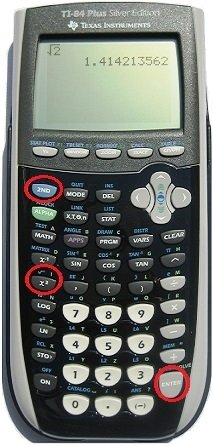## Lesson 4: Estimating Square Roots

When the answer to a square root expression is not a whole number, you can estimate by comparing to the closest square numbers.

Reminder: Examples of square numbers include: $$25 \; (5^2), 81 \; (9^2), 100 \; (10^2)$$ and $$169 \; (13^2)$$

To estimate the square root of 10, find the closest perfect square:

 $$\textcolor{blue}{9}$$ $$\textcolor{green}{10}$$ $$\textcolor{orange}{16}$$

Now take the square root:

 $$\textcolor{blue}{\sqrt{9}}$$ $$\textcolor{green}{\sqrt{10}}$$ $$\textcolor{orange}{\sqrt{16}}$$

The square root of 10 must be in-between 3 and 4:

 $$\textcolor{blue}{3}$$ $$\textcolor{green}{\sqrt{10}}$$ $$\textcolor{orange}{4}$$

Since 10 is closer to 9, approximate the square root of 10 as:

$$\textcolor{green}{\sqrt{10} \approx 3.2}$$

To evaluate the square root of a non-square number, use the square root button on your calculator:Estimate the square root before you use your calculator. Since 24 is just smaller than 25 and the square root of 25 is 5, the square root of 24 should be around 4.9.

$$\sqrt{24} = 4.898979$$

Estimate the square root: